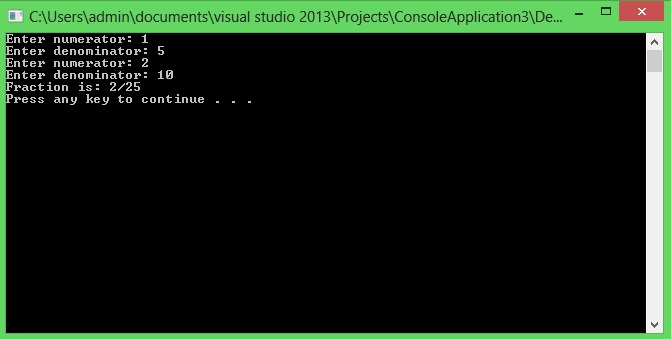# Friend functions

In this part you will learn: 1. What are friend functions 2. Uses of friend function 3. Syntax of friend function 4. Program 5. Output Friend Function A function declared as friend function isn’t really a part of member functions of a class but it can access the public as well as the private members of the class which considers the function as its friend. There are three most common uses of friend function. • To access member variables of two different classes to perform operations on it. • Making one class friend of the other to allow access to class members (private and protected). • To perform function on class without specifying the caller function. Right now we will consider the first and the second, the last one will be discussed in detail in the coming topic of operator overloading. Syntax Following is the syntax of making a function friend of a class. class Class_Name { friend return_type Function_name(parameters without variable names); } return_type Function_name(parameters) { //function definition } As we can see in the syntax that the function which is going to become a friend of class is declared/defined outside the class, the only part present in the class is its prototype following label “friend”. Note: while declaring a function friend in class, we don’t write the names of variables being passed in the function, i.e. we just write the data_type of parameters being passed in the function. By the example given below the concept will become clear. Program with Friend function In this example I have declared a Friend function “gcd” which calculates and returns the greatest common divisor of the fraction passed in it. Basic Step:
`#include<iostream>#include<conio.h>using namespace std;`
These two are the most common and basic lines of a c++ program. The first one iostream is used to grant us most of the basic functions of C++ like Input and Output functions. The second one conio.h means console input output, the black screen we see is the console. Using namespace std; provides us an ease of using all the library functions without specifying that this function belongs to standard library. Without including it, we have to write ‘std’ with all the built-in functions. Class
`class fraction{private:			//access specifier 				int num;	int denom;public:	void getfraction(){		cout << "Fraction is: " << num << "/" << denom << endl;		}	void setfraction(){		cout << "Enter numerator: ";		cin >> num;		cout << "Enter denominator: ";		cin >> denom;		}	void setnum(int n){		num = n;		}	void setdenom(int d){		denom = d;		}	int getnum(){		return num;		}	int getdenom(){		return denom;		}	fraction addfraction(fraction f){		fraction sum;		sum.num = (this->num*f.denom) + (this->denom*f.num);		sum.denom = this->denom*f.denom; 		return sum;		}	friend int gcd(fraction); };`
The above code is the definition of class. We have two private data members num and denum. In member functions we have getter and setter of whole fraction class. Then two setters and getters each to access the private data members. A function addfunction to add two fractions. Last we have a friend function gcd in which an object of class fraction is passed as parameter and it returns the greatest common divisor of the passed fraction type object. Since it is a friend function, so its implementation is outside the definition of class. The definition of function “gcd” is given below. Friend Function Defintion
`int gcd(fraction f){	int temp;				//variable declaration	if (f.num != 0)			//condition when numerator is not equal to zero	{		while (f.num != 0)		{			temp = f.denom%f.num;	//calculations			f.denom = f.num;		//swaping			f.num = temp;		//swaping		}	}return f.denom;}`
Note that the private members of class “fraction” are being directly accessed in the function “gcd”, this is because this function is declared the friend of class “fraction”, so it can access the private (or protected) members too. Following is the main part of the program. Main
`int main(){	fraction f1;		//initializing a variable f1 of type fraction	fraction f2;		//initializing a variable f2 of type fraction	f1.setfraction();	f2.setfraction(); 	fraction f3;	f3 = f1.addfraction(f2); 	f3.setnum(f3.getnum() / gcd(f3));	f3.setdenom(f3.getdenom() / gcd(f3)); 	f3.getfraction(); 	system("pause");}`
In the main function first we initialized two objects of class “fraction”, then we assigned values to them. After that we made another object “f3” in which we stored the sum of two fractions f1 and f2. Then comes the use of our friend function. “f3.setnum(f3.getnum() / gcd(f3));” In this statement we are calling two different functions in the parameter of a function. i.e. we are calling the function setnum through object f3 and passing the value obtained by dividing the value returned by getnum for object f3 with the value returned by function gcd as a parameter. In simple words we are dividing “num” of object f3 by the greatest common divisor obtained by the friend function gcd and storing the updated value back in the member variable “num” of object f3. Same goes for denom and then the fraction f3 will be printed in the lowest form. Output